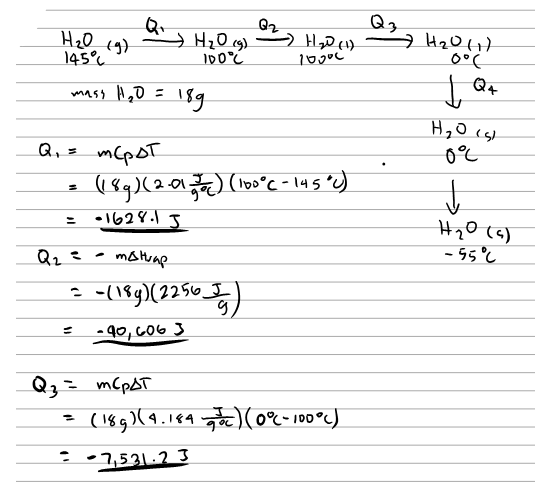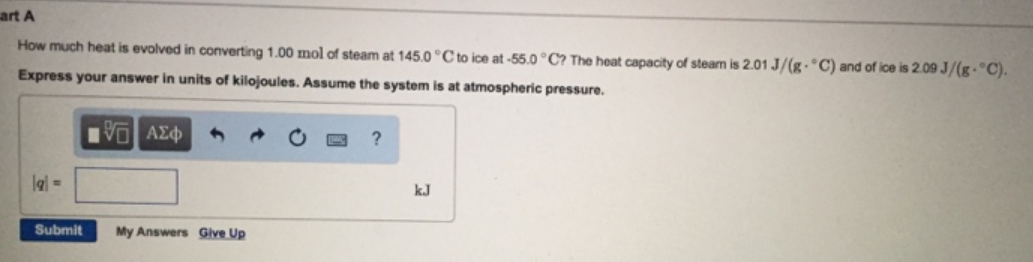# Problem: Part AHow much heat is evolved in converting 1.00 mol of steam at 145°C to ice at 55.0°C? The heat capacity of steam is 2.01 J/(g°C) and of ice is 2.09 J/(g°C). Express your answer in units of kilojoules. Assume the system is at atmospheric pressure.

###### FREE Expert Solution###### Problem Details

Part A

How much heat is evolved in converting 1.00 mol of steam at 145°C to ice at 55.0°C? The heat capacity of steam is 2.01 J/(g°C) and of ice is 2.09 J/(g°C).

Express your answer in units of kilojoules. Assume the system is at atmospheric pressure.